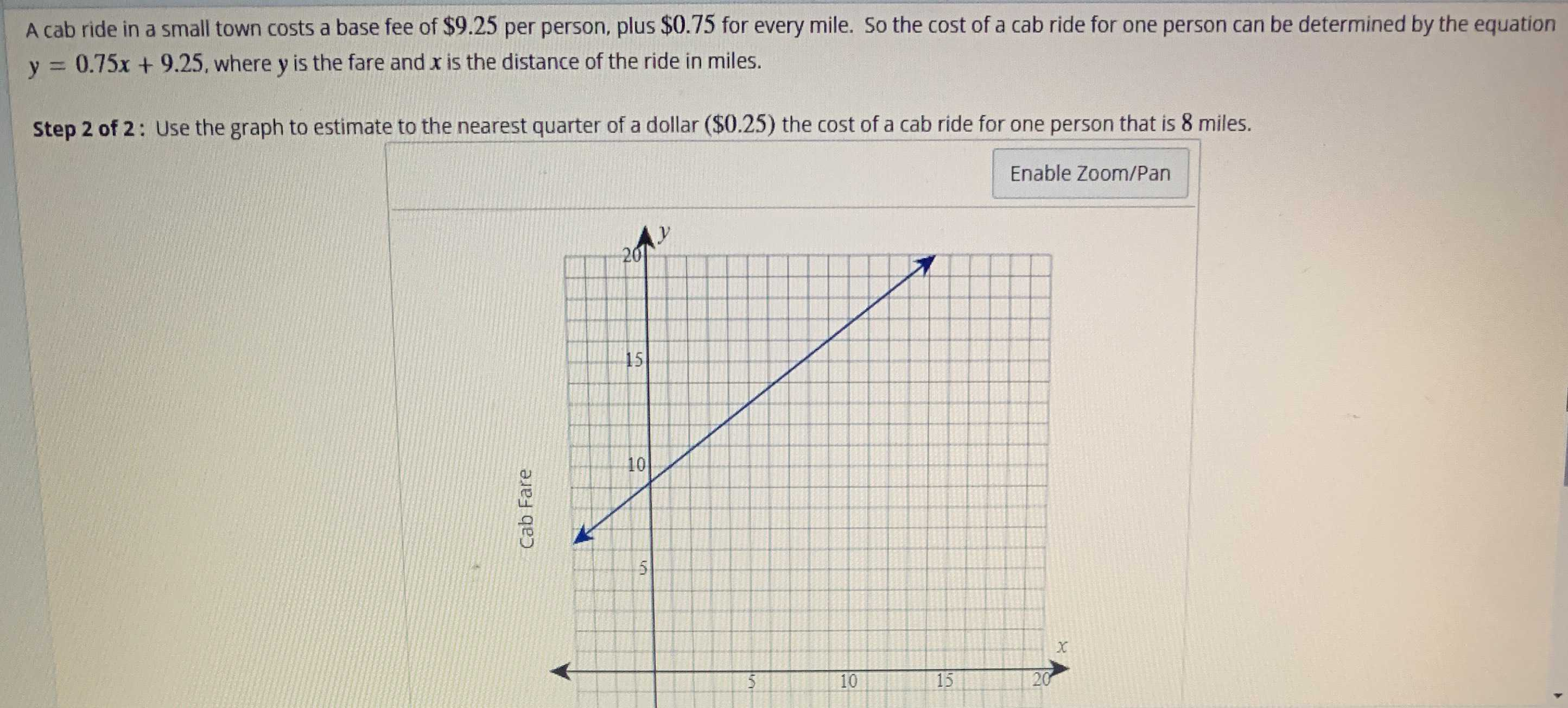### ¿Todavía tienes preguntas de matemáticas?

Pregunte a nuestros tutores expertos
Algebra
PreguntaA cab ride in a small town costs a base fee of $$\ 9.25$$ per person, plus $$\ 0.75$$ for every mile. So the cost of a cab ride for one person can be determined by the equation $$y = 0.75 x + 9.25$$ , where $$y$$ is the fare and $$x$$ is the distance of the ride in miles.

Step $$2$$ of $$2$$ : Use the graph to estimate to the nearest quarter of a dollar $$( \ 0.25 )$$ the cost of a cab ride for one person that is $$8$$ miles.